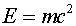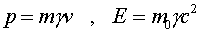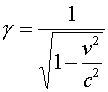Momentum and energy Perhaps the most famous expression in physics isThis expresses the fact that an object at rest has energy. This expression becomes slightly different for moving objects. Consider an object whose mass would be m0 if it were not moving. A moving object has an energy and momentum given bywhere g is the same factor used previously.Note that as the velocity approaches c that both the momentum and energy can become infinite. Thus, even though an object's velocity is constrained to be less than c, the object's momentum and energy are not bounded.